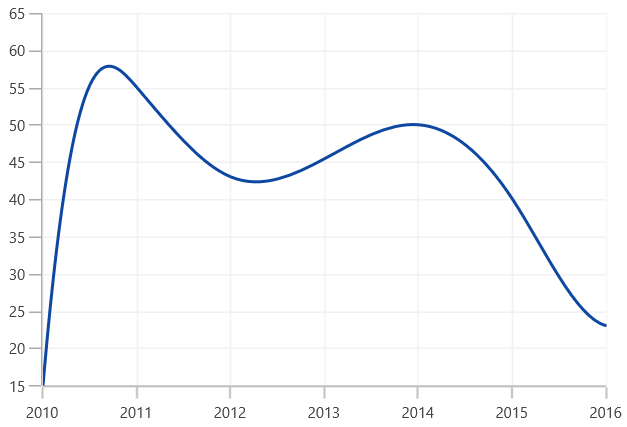# Spline in WinUI Chart

27 May 20224 minutes to read

## Spline

`SplineSeries` resembles line series, but the difference between them is that instead of connecting the data points with line segments, the data points are connected by smooth Bezier curves.

``````<chart:SplineSeries XBindingPath="Year" ItemsSource="{Binding Data}" YBindingPath="Value1"/>

<chart:SplineSeries XBindingPath="Year" ItemsSource="{Binding Data}" YBindingPath="Value2"/>``````
``````SplineSeries series1 = new SplineSeries()
{

ItemsSource = new ViewModel().Data,

XBindingPath = "Year",

YBindingPath = "Value1",

};

SplineSeries series2 = new SplineSeries()
{

ItemsSource = new ViewModel().Data,

XBindingPath = "Year",

YBindingPath = "Value2",

};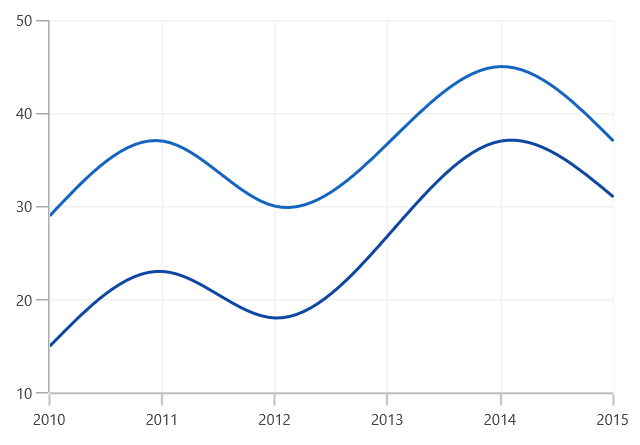Dashed Lines

The `StrokeDashArray` property of the `SplineSeries` is used to render spline series with dashes.

``````<chart:SplineSeries XBindingPath="Year" ItemsSource="{Binding Data}" YBindingPath="Value1" StrokeDashArray="5,3"/>

<chart:SplineSeries XBindingPath="Year" ItemsSource="{Binding Data}" YBindingPath="Value2" StrokeDashArray="5,3"/>``````
``````SplineSeries series1 = new SplineSeries()
{

ItemsSource = new ViewModel().Data,

XBindingPath = "Year",

YBindingPath = "Value1",

};

SplineSeries series2 = new SplineSeries()
{

ItemsSource = new ViewModel().Data,

XBindingPath = "Year",

YBindingPath = "Value2",

};

DoubleCollection doubleCollection = new DoubleCollection();

series1.StrokeDashArray = doubleCollection;
series2.StrokeDashArray = doubleCollection;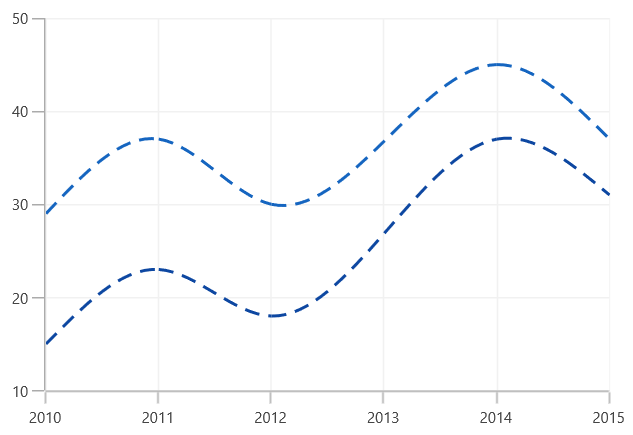## Spline Area

`SplineAreaSeries` connects a series of data points using smooth Bezier line curves, with the underlying areas filled.

``<chart:SplineAreaSeries ItemsSource="{Binding Products}" XBindingPath="ProdName" YBindingPath="Price"/>``
``````SplineAreaSeries series = new SplineAreaSeries()
{

ItemsSource = new ViewModel().Products,

XBindingPath = "ProdName",

YBindingPath = "Price",

};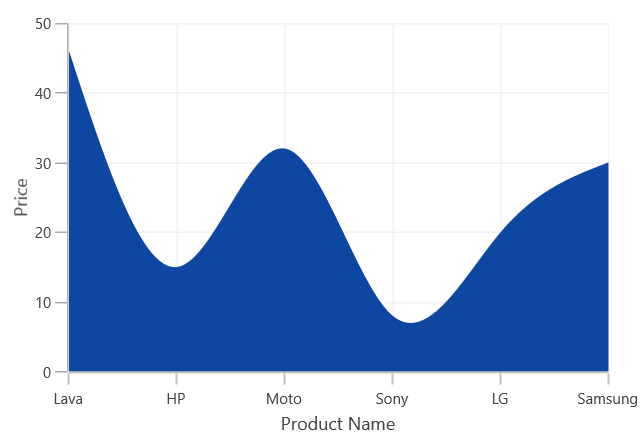## SplineType

The `Spline` and `SplineArea` series support various spline types. The spline type of the series can be changed by using the `SplineType` property. The following spline types are supported by Spline and SplineArea series:

``````   * Natural
* Monotonic
* Cardinal
* Clamped
``````

Natural

The following code illustrates how to set the `SplineType` value as `Natural`.

``````<chart:SplineSeries SplineType="Natural">

</chart:SplineSeries>``````
``````SplineSeries series = new SplineSeries();

series.SplineType = SplineType.Natural;``````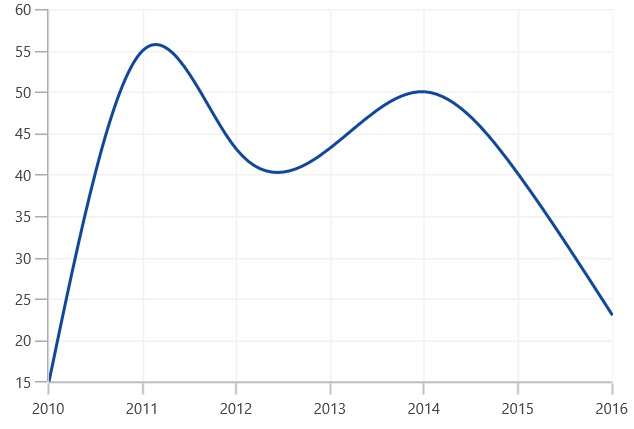Cardinal

The following code illustrates how to set the `SplineType` value as `Cardinal`.

``````<chart:SplineSeries SplineType="Cardinal">

</chart:SplineSeries>``````
``````SplineSeries series = new SplineSeries();

series.SplineType = SplineType.Cardinal;``````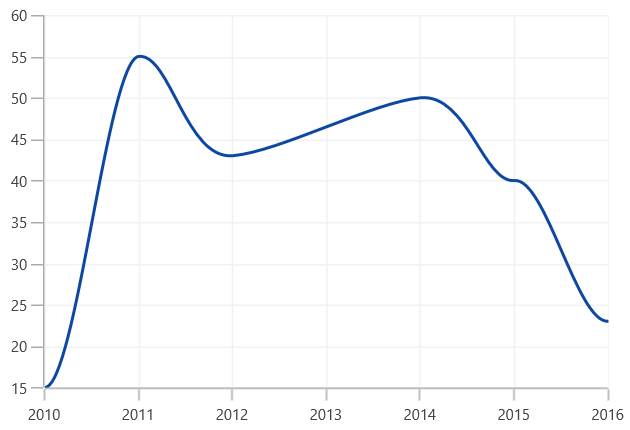Monotonic

The following code illustrates how to set the `SplineType` value as Monotonic.

``````<chart:SplineSeries SplineType="Monotonic">

</chart:SplineSeries>``````
``````SplineSeries series = new SplineSeries();

series.SplineType = SplineType.Monotonic;``````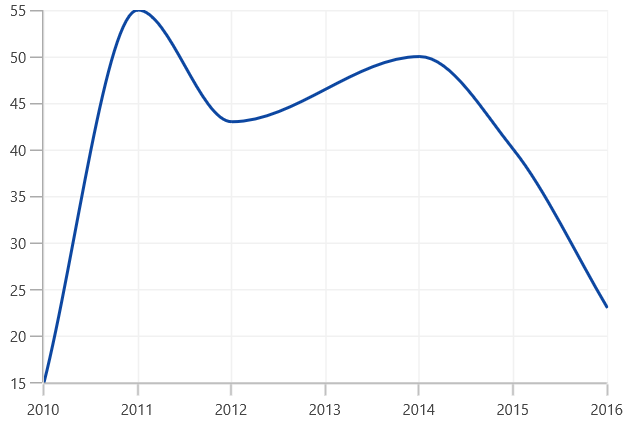Clamped

The following code illustrates how to set the `SplineType` value as Clamped.

``````<chart:SplineSeries SplineType="Clamped">

</chart:SplineSeries>``````
``````SplineSeries series = new SplineSeries();

series.SplineType = SplineType.Clamped;``````